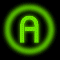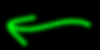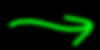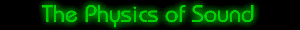There are four main parts to a sound wave: wavelength, period, amplitude, and frequency. In this section, we will discuss each one of these parts. Also, we will talk about pitch and its relation to the frequency of a sound wave.Wavelength and Period:The wavelength is the horizontal distance between any two successive equivalent points on the wave. That means that the wavelength is the horizontal length of one cycle of the wave. The period of a wave is the time required for one complete cycle of the wave to pass by a point. So, the period is the amount of time it takes for a wave to travel a distance of one wavelength.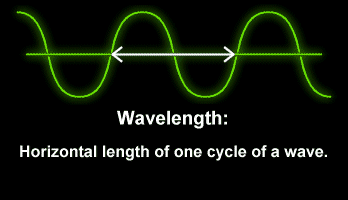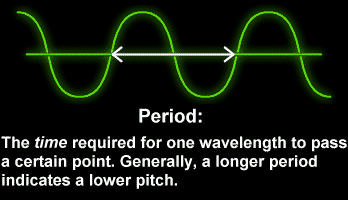Amplitude:The amplitude of a sound is represented by the height of the wave. When there is a loud sound, the wave is high and the amplitude is large. Conversely, a smaller amplitude represents a softer sound. A decibel is a scientific unit that measures the intensity of sounds. The softest sound that a human can hear is the zero point. When the sound is twice as loud, the decibel level goes up by six. Humans speak normally at 60 decibels.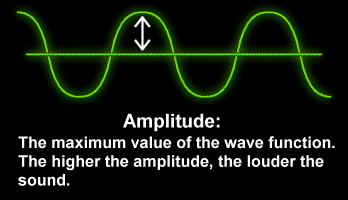Frequency:Every cycle of sound has one condensation, a region of increased pressure, and one rarefaction, a region where air pressure is slightly less than normal. The frequency of a sound wave is measured in hertz. Hertz (Hz) indicate the number of cycles per second that pass a given location. If a speaker's diaphragm is vibrating back and forth at a frequency of 900 Hz, then 900 condensations are generated every second, each followed by a rarefaction, forming a sound wave whose frequency is 900 Hz.

Pitch:How the brain interprets the frequency of an emitted sound is called the pitch. We already know that the number of sound waves passing a point per second is the frequency. The faster the vibrations the emitted sound makes (or the higher the frequency), the higher the pitch. Therefore, when the frequency is low, the sound is lower.